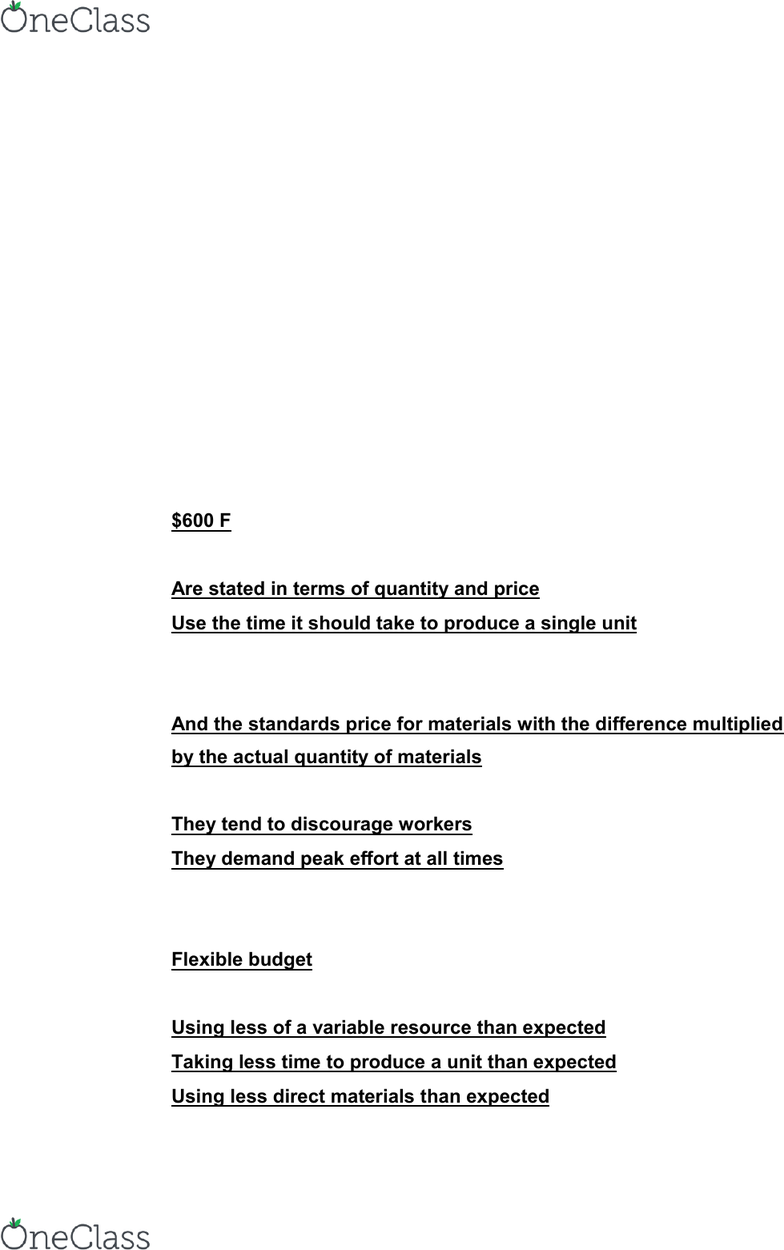Textbook Notes (290,000)
US (110,000)
LSU (20,000)
ACCT (600)
ACCT 2101 (100)
Chapter 9

# ACCT 2101 Chapter Notes - Chapter 9: Standard Cost Accounting, Variable Cost, Cost Driver

Department
Accounting
Course Code
ACCT 2101
Professor
juliechenier
Chapter
9

Page:
of 5LearnSmart Chapter 9: Standard Costing and Variances
TRUE/FALSE
1. The variable overhead rate variance uses the same basic formula as the
labor rate variance except that the variable overhead rates are used instead
of the direct labor rates: TRUE
2. Quantity standards refer to the price to be paid for each unit of input: FALSE
3. A favorable material price variance is always a good thing: FALSE
4. Favorable variances always indicate good performance: FALSE
MULTIPLE CHOICE
5. The standard price of materials is \$4.10 per pound and the standard quantity
allowed for actual output 5,800 pounds. If the actual quantity purchased and
used was 6,000 pounds, and the actual price per pound was \$4.00, the direct
materials price variance is:
\$600 F (6,000x(4.00-4.10)=\$600)
6. Direct labor standards:
Are stated in terms of quantity and price
Use the time it should take to produce a single unit
7. The direct materials price variance is the difference between the actual price
of materials:
And the standards price for materials with the difference multiplied
by the actual quantity of materials
8. Ideal standards are problematic because:
They tend to discourage workers
They demand peak effort at all times
9. Which of the following shows how budgeted costs and revenues will change
over different volumes?
Flexible budget
10. Which of the following are common causes of favorable variances?
Using less of a variable resource than expected
Taking less time to produce a unit than expected
Using less direct materials than expected
find more resources at oneclass.com
find more resources at oneclass.com
LearnSmart Chapter 9: Standard Costing and Variances
11. Using the information provided, calculate the direct materials quantity
variance:
Standard Price
\$3.00 per pound
Actual Price
\$3.20 per pound
Actual quantity used
5,200 pounds
Standard quantity allowed
5.000 pounds
\$600 Unfavorable (SP(AQ-SQ)=3.00(5,200-5,000)=\$600 Unfavorable)
12. Given the following information, calculate the variable overhead efficiency
variance.
Actual Hours
1,500
Standard hours allowed
1,350
\$3.00 per hour
\$3.50 per hour
\$525 Unfavorable (When actual hours are greater than standard hours, the
variable overhead efficiency variance is unfavorable. (1,350-1,500) x \$3.50
=\$525)
13. If the planned fixed overhead for 5,000 units is \$120,000, what is the flexible
budget fixed overhead for 4,500 units?
\$120,000 (budgeted fixed costs do not change within the relevant range)
14. Unfavorable labor rate variances may occur as a result of:
Skilled workers being assigned to jobs requiring little skill
Overtime premiums being charged to the direct labor account
15. An unfavorable variance may be caused by:
Paying more than expected
16. Which of the following statements are true?
Both the master budget and the flexible budget are based on
standard costs
The flexible budget can be used as a benchmark for evaluating
performance
The master budget is a static budget
find more resources at oneclass.com
find more resources at oneclass.com
LearnSmart Chapter 9: Standard Costing and Variances
17. Which of the following statements are true?
Companies generally try to hold specific managers responsible for
specific volumes
Variances always compare actual results to budgeted or standard
results
When calculating variances only one factor changes at a time
18. Price variance is the difference between the:
Actual price and the standard price multiplied by the actual amount
of the input
19. Direct labor standards:
Are stated in terms of quantity and price
Use the time it should take to produce a single unit
20. The materials price variance is calculated using the:
Actual price of the input
Actual quantity of the input purchased
Standard price of the input
21. Use the following information to calculate the direct labor rate variance for
Actual hours used
5,500
Standard hours allowed
5,800
Actual labor rate
\$14.75 per hour
Standard labor rate
\$14.00 per hour
\$4,125 Unfavorable (the direct labor rate variance is: AH(AR-SR): 5,500 x
(\$14.75-14.00) = \$4,125)
22. Poor supervision is one possible cause of an unfavorable ____ variance
Direct labor efficiency
find more resources at oneclass.com
find more resources at oneclass.com